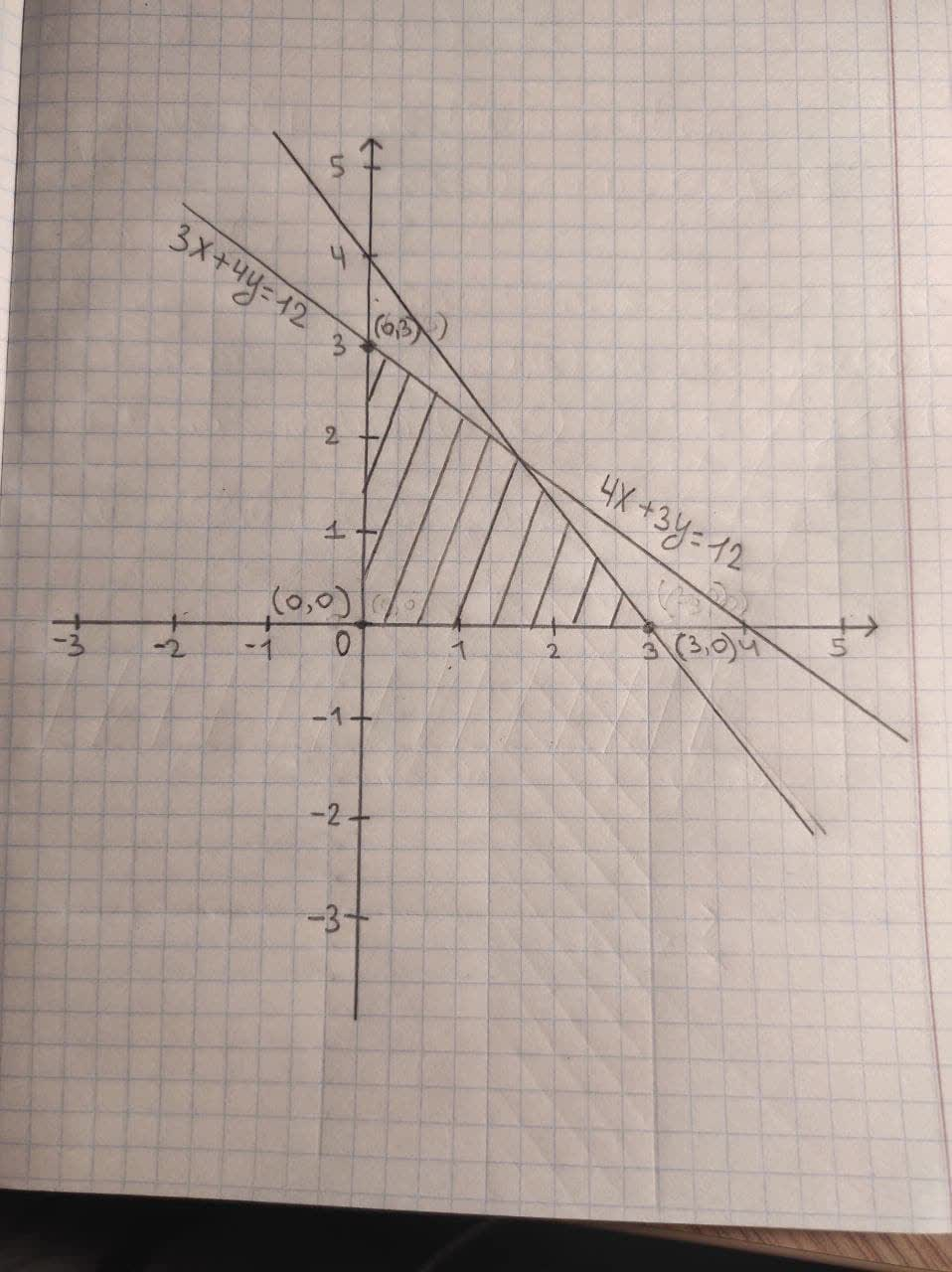Question# Consider the following system of linear inequalities. 3x+4y <=12 4x+3y <= 12 x >= 0 y >= 0 graph, shade and find corner points.

Inequalities systems and graphs
ANSWEREDConsider the following system of linear inequalities.
$$\displaystyle{3}{x}+{4}{y}\le{12}$$
$$\displaystyle{4}{x}+{3}{y}\le{12}$$
$$\displaystyle{x}\ge{0}$$
$$\displaystyle{y}\ge{0}$$
graph, shade and find corner points.